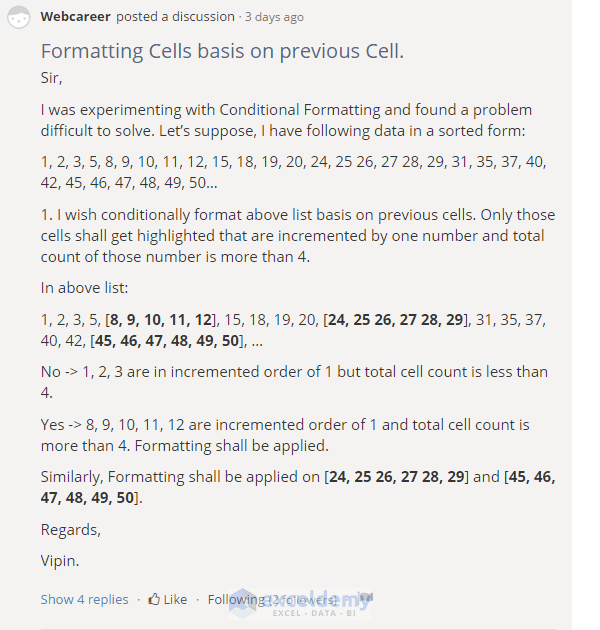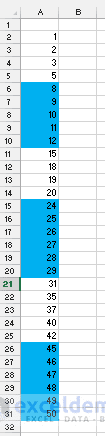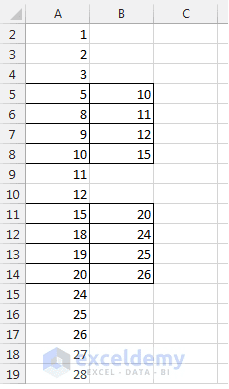# How to Make FOR LOOP in Excel Using Formulas?

Don’t you want to use Excel VBA and want to make a FOR LOOP in Excel using Formulas?

In this article, I’ve shown how you can make one using functions: OFFSET(), MAX() and MIN()!

If you know how to code with Excel VBA, you’re blessed 🙂

If you never wrote a code in VBA or want to keep your Excel workbook free of Excel VBA code, then you know: To create a simple loop you have to think out of the box most of the time.

Let me know you the background that is encouraging me to write this blog post.

I am the author of some courses in Udemy. My one of the courses is on Excel Conditional Formatting. The course title is: Learn Excel Conditional Formatting with 7 Practical Problems [to get free access to this course, click here].

In the course discussion board, a student asked me a question as below [screenshot image].Question asked by a student in Udemy.

Read the above question carefully and try to solve it…

Here are the steps to solve the above problem:

1. Your job is: open a new workbook and input the above values one by one into the worksheet [start from cell A2].
2. If you’re done, just select the whole range [from cell A2: A31].
3. Click on the Conditional Formatting drop down in the Home ribbon, select New Rule from the drop-down, New Formatting Rule dialog box appears.
4. In the Select a Rule Type window, select Use a formula to determine which cells to format option.
5. In the Format values where this formula is true field, type this formula: =OR(OFFSET(A2,MAX(ROW(A\$2)-ROW(A2)+3,0),0,MIN(ROW(A2)-ROW(A\$2)+1,4),1)-OFFSET(A2,MAX(ROW(\$A\$2)-ROW(A2),-3),0,MIN(ROW(A2)-ROW(A\$2)+1,4),1)=3)
6. Now select the appropriate format type clicking on the Format… button in the dialog box [I selected Sky Blue background].
7. Now click OK for two times, you’re done! You get the cells conditionally formatted like the following image.Conditionally Formatted Cells.

My motto to write this post is not to show you how to solve the above problem. The motto is: how to make a loop in Excel using 3 Excel functions: OFFSET, MAX, and MIN. You can apply this technique to any loop related Excel formulas.

Let me show you the algorithm to solve the above problem:

1. To make you understand the algorithm easily, I will explain the whole thing with two reference cells: cell A8 and A14. In cell A8 and A14, the values are 10 and 20 respectively (above image). If you are used to with Excel formulas, then you can smell the OFFSET function, as OFFSET function works with reference points.
2. Imagine I am taking the values of cell ranges A5: A8 & A8: A11, and A11: A14 & A14: A17 side by side [image below]. Reference cells are A8 and A14 and I am taking total 7 cells around the reference cell. You will get an imaginary picture like the following. From the first part, you can find a pattern from the image. A6 – A9=3, A7-A10=3, there is a pattern. But for the second part, there is no such pattern.A5: A8 and A8: A11
and
A11: A14 and A14: A17 side by side.

3. So let’s build the algorithm with the above pattern keeping in mind. Before building the common formula, I shall show what the formulas will be for the cells A8 and A14 and then will modify the formula to make it common for all. For a reference point (like A8 or A14), we shall take total 7 cells around it (including the reference point) and place them side by side in the formula creating arrays. Then we shall find out the difference of the arrays if any of the differences is equal to 3 that reference cell will be TRUE valued.
4. We can do that easily using OFFSET function as OFFSET function returns array. Say for cell reference A8, I can write the formula like this: =OR(OFFSET(A8, 0, 0, 4, 1)-OFFSET(A8, -3, 0, 4, 1)=3). What this formula will return? The first offset function of the formula will return array: {10; 11; 12; 15}, second offset function will return array {5; 8; 9; 10}. And you know {10; 11; 12; 15} – {5; 8; 9; 10} = {10-5; 11-8; 12-9; 15-10} = {5; 3; 3; 5}. When this array is logical tested with =3 then Excel calculates internally like this: {5=3; 3=3; 3=3; 5=3} = {False; True; True; False}. When OR function is applied on this array: OR({False; True; False; True}, we get TRUE. So cell A8 gets true values as returned.
5. I think you have got the whole concept how this algorithm is going to work. Now there is a problem. This formula can work from cell A5, above cell A5, there are 3 cells. But for cells A2, A3, and A4 this formula cannot work. So the formula should be modified for these cells.
6. For cells A2 to A4, we want that the formula will not take into considerations the upper 3 cells. For example, for cell A3, our formula will not be like the formula for cell A8: =OR(OFFSET(A8, 0, 0, 4, 1)-OFFSET(A8, -3, 0, 4, 1)=3).
7. For cell A2, the formula will be like: =OR(OFFSET(A2, 3, 0, 1, 1)-OFFSET(A2, 0, 0, 1, 1)=3).
8. For cell A3, the formula will be like: =OR(OFFSET(A3, 2, 0, 2, 1)-OFFSET(A3, -1, 0, 2, 1)=3).
9. For cell A4, the formula will be like: =OR(OFFSET(A4, 1, 0, 3, 1)-OFFSET(A3, -2, 0, 3, 1)=3).
10. For cell A5, the formula will be like: =OR(OFFSET(A5, 0, 0, 4, 1)-OFFSET(A5,-3, 0, 4, 1)=3); [this is the general formula]
11. For cell A6, the formula will be like: =OR(OFFSET(A6, 0, 0, 4, 1)-OFFSET(A6,-3, 0, 4, 1)=3); [this is the general formula]
12. Do you find some patterns from the above formulas? The first Offset function’s rows argument has decreased from 3 to 0; height argument has increased from 1 to 4. Second Offset function’s rows argument has decreased from 0 to -3 and height argument has increased from 1 to 4.
13. First Offset function’s rows argument will be modified like this: MAX(ROW(A\$2)-ROW(A2)+3,0)
14. Second Offset function’s rows argument will be modified like this: MAX(ROW(\$A\$2)-ROW(A2),-3)
15. First Offset function’s height argument will be modified like this: MIN(ROW(A2)-ROW(A\$2)+1,4)
16. Second Offset function’s height argument will be modified like this: MIN(ROW(A2)-ROW(A\$2)+1,4)
17. Try to understand the above modification. These are not that tough to understand. All these four modifications are working as FOR LOOPS of Excel VBA but I’ve built them with Excel Formulas.
18. So you got the ways how the general formula works for the cells from A2: A31.

So we were talking about Looping in Excel spreadsheet. This is a perfect example of looping in Excel. Every time the formula takes 7 cells and works on the cells to find out a specific value.

If you have any feedback to add to this post, please feel free to put it in the comment box below.

How to Use the Do While Loop in Excel VBA

For Next Loop in VBA Excel (How to Step and Exit Loop)

Make-Loop-Using-Offset-Max-and-Min-Functions.xlsx

#### Kawser

Hello! Welcome to my Excel blog! It took me some time to be a fan of Excel. But now I am a die-hard fan of MS Excel. I learn new ways of doing things with Excel and share here. Not only how to guide on Excel, but you will get also topics on Finance, Statistics, Data Analysis, and BI. Stay tuned!

Karthik August 25, 2016 at 6:38 AM

Hi Kawser,

nice post. However, I couldn’t solve this loop using offset.. Any ideas?
what i’m trying to do is add the letter “A” in front of the string “BCD” until length of the string becomes 10. (for which A would need to be added 7 times in front of “BCD” to make it “AAAAAAABCD”

Kawser August 25, 2016 at 10:02 AM

If cell A1 holds your string “BCD” and you want to show “AAAAAAABCD” in cell B1, input this formula in cell B1: =REPT(“A”, 10-LEN(A1))&A1.
To solve this problem, you don’t have to use any looping. Or, do you have some other requirement for this problem? Let me know.
Best regards.

Bijan April 14, 2018 at 4:52 AM

hi,
Thank you for details information.
I have 2 columns of numbers , say each column 10 numbers , total 20 numbers.
I want delete the similar numbers in both columns and keep just the numbers are different. Numbers need be sorted from small to large too.
this is urgent case.

Any comment I appreciate.

January 11, 2019 at 10:56 AM

can i know how can i make the rows change as per the set values. Ex:
If i entered 5, rows in excel=5 & if 6, rows in excel=6 & so on…

JEFF April 28, 2019 at 8:45 AM

Great formula but I can’t understand how to incorporate it into a SUMIFS formula that I need to perform a loop.